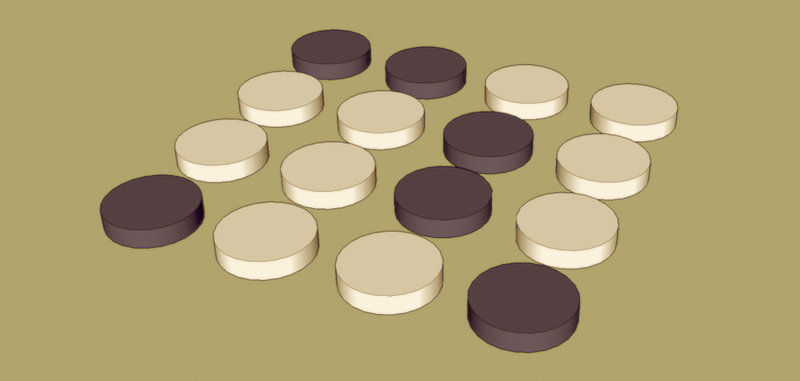# A 2D Nim Game### Intro

• There are 16 stones, arranged in a 4 row * 4 column grid.
• Each player take stone(s) in turn.
• Once the stone is taken, there is a gap on it’s original location on the grid.
• In each take, one should take no more than 3 stones (1/2/3). Only those stones in the same row or in the same column without gap between them could be taken.
• Player who takes the last stone LOSE

Let me demonstrate it. Assuming 1 means there is stone on the specific cell and 0 represent a gap in the following chart. And those state that would definitely lose are called Losing State.

• the initial state could be represented as (I)
• the ending state could be represented as (II)
• one of the losing state (LS) for current turn player could be represented as (III)

To be more specific, the following state is a possible game.

### Initial analysis

According to my primary school playing experience, all of the following states are LS (and welcome to try).

Believe it or not, knowing this states make you 99% unbeatable in primary school.

But I want to discover the essence of this game. So let’s do some deep dive in 20 years later.

Instead of staying on the number axis, such as some basic Nim game subtraction problem) , this game is in a 2D space, and there are a lot of variation in each take. The first thought come to me is that this game is about graph theory and the state analysis becoming connected graph analysis since removing stones require connectivity. After a simple estimation of my poor graph theory knowledge, I give up this direction shamelessly and try to analyse the state space of this game.

Thanks to the simple nature of this game, there are only 2^16 = 65536 states in this game.

And we know that the following 16 states are absolutely going to lose the game. If we could leave this 16 states to our opponent, then we could win the game.

So any state that transfer to these 16 states in one move, will be our winning state. Because if we get any of those states, then we have at least one way to transfer this state to the above 16 lose states for our opponent. For example, these states are some of the winning state towards the first state in above situations.

After calculate all such states, we can easily get 600+ win states.

Great, but how to proceed? Any state could transfer to a win-state in one move is lose-state? Not necessarily, check the first 2 states: 1100 and 1110, in the above graph. 1110 can transfer to 1100 but it still win because we could choose to leave only 1000 to our opponent.

But it give us a hint for the deduction for two smart enough player,

if ANY next state of current state LOSE, current state WIN

And with further consideration,

Only ALL next state(s) of current state WIN, current state LOSE

Let’s do a quick test with ZERO state. If a player get this state, means his opponent take the last stones, so ZERO state is a win state. The previous 16 states have only 1 next state, the ZERO state. So they are indeed the lose state.

Our game is a typical case of zero-sum perfect-information game and it match the Zermelo’s Theorem). In such a game, for any state, one side of the game will have a series of strategy that could definitely win the game. So it also mean any state in the game will be either a win-state or a lose-state.

Seems we are making progress, but we still need a way to expand the lose-state / win-state set. This game will be conquered once all 65536 states are figured out.

### Solution generation

With the support of Zermelo, and the previous deduction that:

if ANY next state of current state LOSE, current state WIN. else, current state LOSE

we are able design the following algorithm to figure out and expand the states set from ZERO state.

First, define a binary notation for a state. For example, the following state could be converted as (1111000011111111)2 = 61695

In this notation, for any state S and all its next states N, (S)2 > (N)2, because we are taking one or more 1 from S to get N.

According to deduction mentioned, if the win/lose state of S’s all next states are known, then the win/lose state of S could be figure out. It’s a quite straight forward problem that could be solved by dynamic programming – After we initialize ZERO state as winning state, we could iterate the whole state space.

The upper bound of count for next states is smaller than 4*4*2*3, because for any possible cell, one could at most remove 3 types of length for 2 directions (down / right)

To be more generalize, for an N*N Grid with above rules, this algorithm take O(2^(N*N)*2*3*N*N) to iterate all possible states. If N = 4, it takes 30 seconds to generate all states on my computer. so it might take much more for N = 5.

If you want to know whether the first player or the second player could force a win, checkout the game script I wrote on github: 2d-nim and test it!

### Summary

We have discussed the 2d nim game, and use Zermelo’s Theorem and Dynamic Programming to get the ultimate strategy for this game. Although we reached the fact of the game, I still believe that there are some topology based solutions with a lower complexity for larger board.

Share